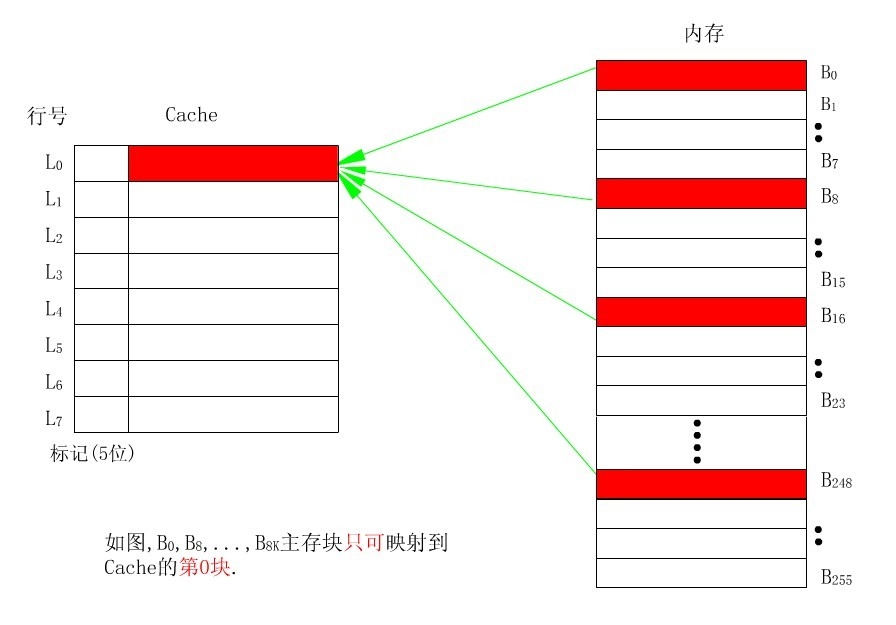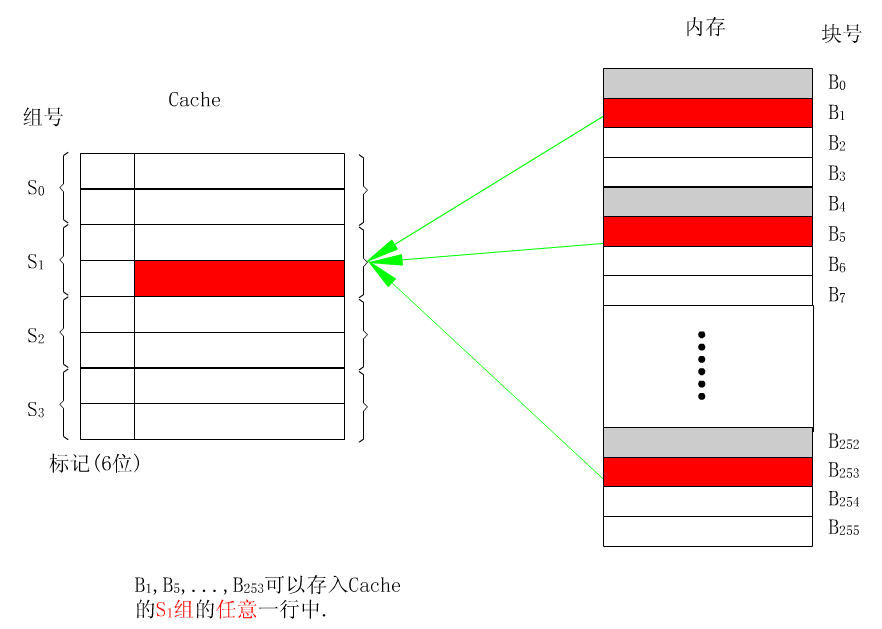# 存储器层次结构（读书笔记）

cache与主存连接的方式有多种：直接映射高速缓存，组相连映射高速缓存。``````
int sumarrayrows(int a[M][M])
{
int i,j,sum=0;

for(i=0;i< M;i++)
for(j=0;j< M;j++)
sum+=a[i][j];
//sum += a[j][i];
return sum;
}
``````